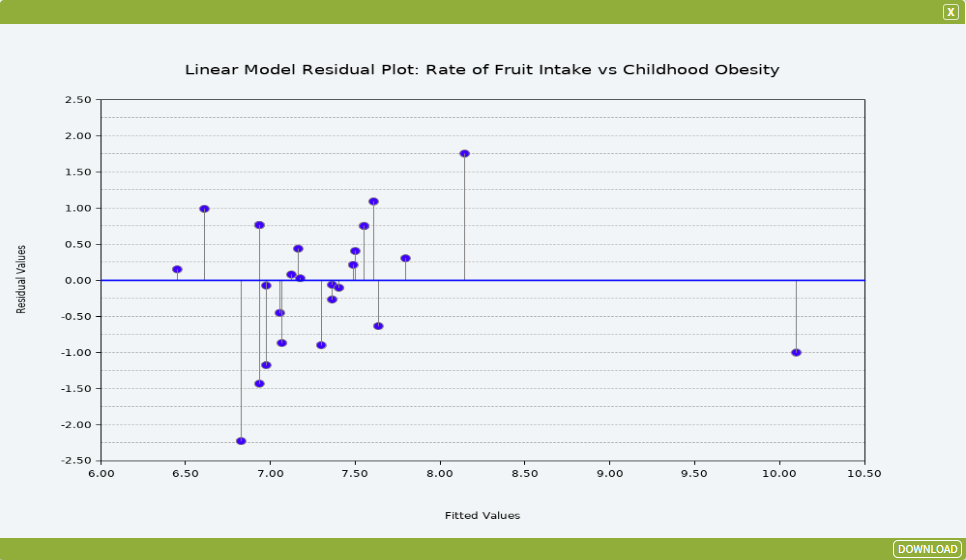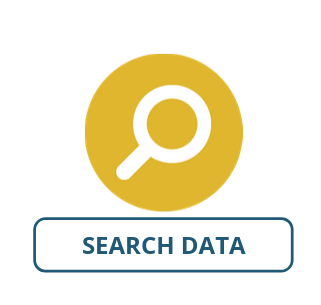# Linear Model Residual Plot

The Linear Model Residual Plot tool is a scatter plot of the response (dependent) variable against the linear model (“line of best fit”) calculated by a regression, plotting the residuals (that is, the part of the dependent variable that can’t be explained by the model – the “residual” variation) against fitted values (i.e. the linear model). The Linear Model Residual Plot is a plot similar to the Linear Model Response Plot tool except instead of showing how the response variable (your dependent variable) against the independent variable, with a positive or negative slope, it has the linear model as a horizontal line (b = 0) to show how much variation there is around the line.

### SET UP

To illustrate the Linear Model Residual Plot tool, we will run it on a dataset within Tasmania to visualise the connection between fruit intake and obesity in children. Prepare the context by:

• Select South Australia as your area.
• Select PHIDU – Prevalence of Selected Health Risk Factors – Children and Youth (LGA) 2014-2015 as your dataset with the following attributes:
• LGA Code 2016
• LGA Name 2016
• Estimated Number Of People Aged 4-17 Years With Adequate Fruit Intake (Modelled Estimates) 2014-15 ASR per 100
• Estimated Number Of Children Aged 2-17 Years Who Were Obese (Modelled Estimates)* 2014-15 ASR per 100

Once you have added the dataset and the selected attributes, you are ready to use the Linear Model Residual Plot tool – follow on to learn about the input options.

### Inputs

Once you have set up your data, open the Linear Model Residual Plot tool (Tools → Charts → Linear Model Residual Plot). The input fields are as follows:

• Dataset Input: The dataset containing the variables that you would like to run through the tool. Select PHIDU – Prevalence of Selected Health Risk Factors – Children and Youth (LGA) 2014-2015.
• Formula: This will need to be typed in the manner that is normally incorporated in the R language. This will require typing in the names rather than the titles of the variables. These can be found in the metadata window of the dataset.
When we ‘regress’ a dependent variable on an independent variable in R, this is written as dependent variable ~ independent variable with the tilde symbol (~) representing the statistical function of “regressed on”. For this example Type est_chld_2_17_yrs_obese_2014_15_asr_100 ~ est_ppl_4_17_yrs_adq_frt_intk_2014_15_asr_100.
• Compute Intercept: Checked means we compute the intercept with the y-axis. Tick this box.
• Chart Title: This is where we enter the name that we want to give the resultant plot. Type Linear Model Residual Plot: Rate of Fruit Intake vs Childhood Obesity.
• Show Gridlines: Check this box if you want gridlines for your graph. Tick this box.
• Greyscale: Check this box if you want your graph to be produced in greyscale. Untick this box.

A summary of the inputs to explore our case can be viewed in the image below, once complete click Run Tool.### Outputs

Once your tool has run, click the Display Output button on the pop-up window that appears. You should get a graph which looks like the graph below.This is a linear model residual plot, with the distance of each observed point marked vertically from the horizontally linear model line.

### Looking for Spatial Data?

You can browse the AURIN Data Discovery:### How can you Create Impact?